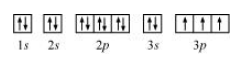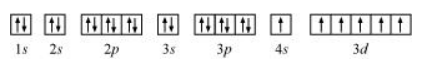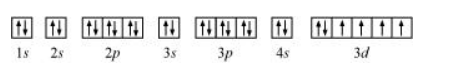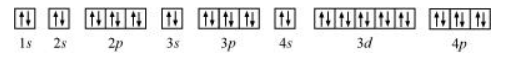# Indicate the number of unpaired electrons in:Question:

Indicate the number of unpaired electrons in: (a) $\mathrm{P}$, (b) $\mathrm{Si}$, (c) $\mathrm{Cr}$, (d) Fe and (e) $\mathrm{Kr}$.

Solution:

(a) Phosphorus (P):

Atomic number $=15$

The electronic configuration of $P$ is:

$1 s^{2} 2 s^{2} 2 p^{6} 3 s^{2} 3 p^{3}$

The orbital picture of $P$ can be represented as:From the orbital picture, phosphorus has three unpaired electrons.

(b) Silicon (Si):

Atomic number = 14

The electronic configuration of Si is:

$1 s^{2} 2 s^{2} 2 p^{6} 3 s^{2} 3 p^{2}$

The orbital picture of chromium is:From the orbital picture, silicon has two unpaired electrons.

(c) Chromium (Cr):

Atomic number $=24$

The electronic configuration of $\mathrm{Cr}$ is:

$1 s^{2} 2 s^{2} 2 p^{6} 3 s^{2} 3 p^{6} 4 s^{1} 3 d^{5}$

The orbital picture of chromium is:From the orbital picture, chromium has six unpaired electrons.

(d) Iron (Fe):

Atomic number $=26$

The electronic configuration is:

$1 s^{2} 2 s^{2} 2 p^{6} 3 s^{2} 3 p^{6} 4 s^{2} 3 d^{6}$

The orbital picture of chromium is:From the orbital picture, iron has four unpaired electrons.

(e) Krypton (Kr):

Atomic number $=36$

The electronic configuration is:

$1 s^{2} 2 s^{2} 2 p^{6} 3 s^{2} 3 p^{6} 4 s^{2} 3 d^{10} 4 p^{6}$

The orbital picture of krypton is:Since all orbitals are fully occupied, there are no unpaired electrons in krypton.